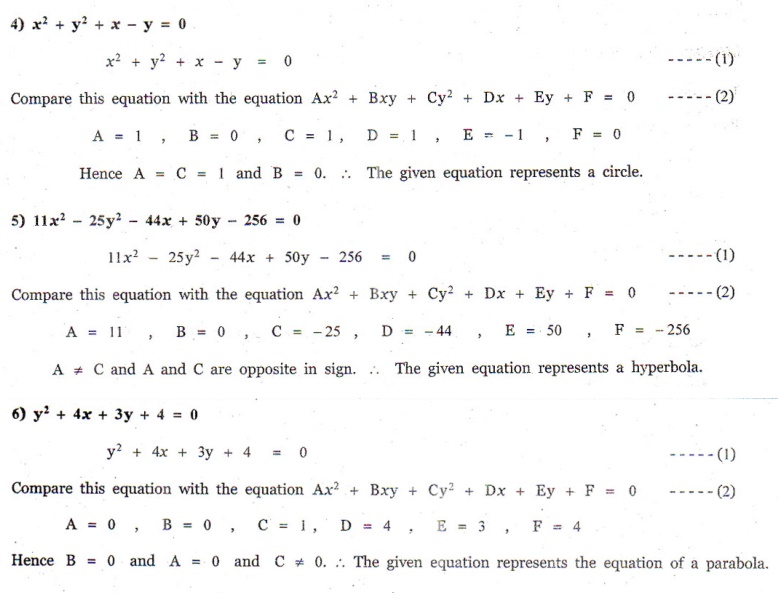Home | | Maths 12th Std | Exercise 5.3: Conic Sections

# Exercise 5.3: Conic Sections

Maths Book back answers and solution for Exercise questions - Identify the type of conic section for each of the equations. Exercise problems with Questions, Answers, Solution, Explanation

EXERCISE 5.3

Identify the type of conic section for each of the equations.

1. 2x2 - y2 = 7

2. 3x2 + 3y2 - 4x + 3y +10 = 0

3. 3x2 + 2y2 = 14

4. x2 + y2 + x - y = 0

5. 11x2 - 25y2 - 44x + 50y - 256 = 0

6. y2 + 4x + 3y + 4 = 01. hyperbola

2. circle

3. ellipse

4. circle

5. hyperbola

6. parabola

Tags : Problem Questions with Answer, Solution , 12th Mathematics : UNIT 5 : Two Dimensional Analytical Geometry II
Study Material, Lecturing Notes, Assignment, Reference, Wiki description explanation, brief detail
12th Mathematics : UNIT 5 : Two Dimensional Analytical Geometry II : Exercise 5.3: Conic Sections | Problem Questions with Answer, Solution